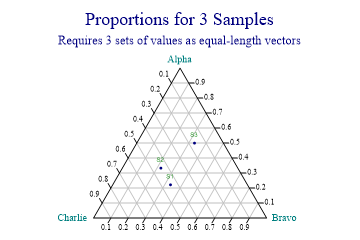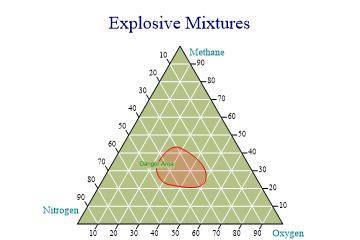SharpPlot Tutorials > Chart Samples > Triangle plots for 3-way proportions

## Triangle plots for 3-way proportions

The Triangle chart is used to analyse data where there are three important constituents, summing to unity. It is common in Geology and Chemical Engineering where a set of 3 key ingredients determines the outcome from some process.

### Plotting the Proportions of 3 values```alpha = new int[] {2,3,3};
bravo = new int[] {3,2,2};
charlie = new int[] {4,4,1};

sp.SetMargins(64,18,0,0);
sp.Heading = "Proportions for 3 Samples";
sp.Subheading = "Requires 3 sets of values as equal-length vectors";

sp.TriangleStyle = (TriangleStyles.Markers|TriangleStyles.Labelled|TriangleStyles.ValueTags|TriangleStyles.GridLines);
sp.XCaption = "Alpha";
sp.YCaption = "Bravo";
sp.ZCaption = "Charlie";
sp.SetValueTags(new string[]{"S1","S2","S3"});
sp.SetMarkers(Marker.Bullet);

sp.DrawTriangle(alpha,bravo,charlie); ```

This chart shows the proportions of 3 constituents found in samples S1,S2 and S3. The allocation of series to chart axes is entirely arbitrary – the convention used is to work around clockwise from 12:00.

The natural data structure for this chart is a 3-column matrix, but an array of 3 data arrays (of equal lengths) is accepted.

### Illustrating a Danger Zone```methane = new int[] {2,3,3,2,2};
oxygen = new int[] {3,2,2,4,3};
nitrogen = new int[] {4,4,2,2,4};

sp.SetMargins(42,12,0,0);

sp.SetPenWidths(1.2);
sp.SetColors(Color.Red);
sp.SetFillStyles(FillStyle.Opacity18);

sp.TriangleStyle = (TriangleStyles.Lines|TriangleStyles.Curves|TriangleStyles.Labelled|
TriangleStyles.ValueTags|TriangleStyles.GridLines|TriangleStyles.Percentage|
TriangleStyles.Filled|TriangleStyles.SideCaptions);

sp.SetChartBackground(ColorTranslator.FromHtml("#B4C287"),FillStyle.Solid,0);
sp.SetGridLineStyle(Color.White,LineStyle.Solid,0.12);
sp.Flexibility = 12;

sp.XCaption = "Methane";
sp.YCaption = "Oxygen";
sp.ZCaption = "Nitrogen";

sp.SetValueTags(new string[]{"","Danger Area"});
sp.ValueTagStyle = (ValueTagStyles.Inside|ValueTagStyles.Opaque);

sp.DrawTriangle(new int[][]{methane,oxygen,nitrogen});```

This is very typical of charts used in the chemical and oil industry, where certain combinations are either desirable or dangerous. Typically a chart such as this would be used as a basis, over which to plot a set of samples.

Note the use of ValueTags (only one is non-null) to label the outline.